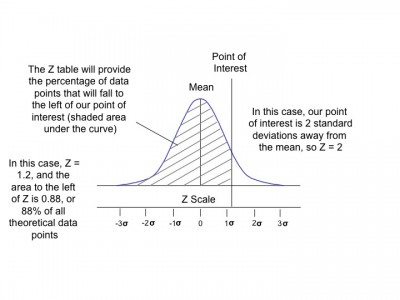# Z Table

When a set of data follows a normal distribution pattern, the mean and standard deviation can be used to calculate the percentage of data falling inside a given range.  The z table makes this possible.

Simple Definition of a Z Score

Referencing the illustration below, the Z score is simply how many standard deviations our point of interest is from the mean of our data set (click on the image to see a full view).Calculating Z

To calculate the percentage of data falling inside a given range, the Z score is calculated using the equation: Z= (x-μ)/σ, where x is the value we are interested in, such as an upper or lower specification limit.

Once the z-score is calculated, a z-table can be referenced to determine the area to the left or right of x.  This area, when multiplied by 100, represents percentage of data to the left or right of x, depending on how we are reading the z-table.